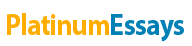# Nike Inc. Case Analysis

By:   •  June 30, 2019  •  Case Study  •  788 Words (4 Pages)  •  1,427 Views

Page 1 of 4

Nike Inc. Case Analysis

This case study analysis is to assist NorthPoint Group in arriving at cost of capital for Nike Inc which will be used as the discount rate for arriving at estimated valuation for Nike Inc’s stock price. Our estimation of cost of capital is 9.27% as detailed in Exhibit 4- WACC of Nike Inc. We have used the WACC model to arrive at this estimation. Further using WACC as the discount rate, we recommend that NorthPoint should buy Nike Inc. as it is currently undervalued.

Cost of Capital and WACC

Weighted average cost of capital is a measure of a firm’s cost of capital that has both debt and equity components in it. Since WACC takes into account the capital structure of the firm, it is an appropriate representative of the expected rate of return by the investors of the company- both equity holders as well as debt holders. Cost of capital is in effect the minimum expected rate of return at which investors will continue to be invested in the company. Since the investors are forgoing other opportunities and have invested in a firm they would expect a certain return on their investment. Further, they would expect to be compensated for the sharing the risks associated with the firm and the industry it is in. Cost of capital measures this expected return and by using the cost of capital as a discount factor, we can effectively establish whether the firm is generating value from its future cash flows and future projects. Firms are always competing for capital and hence it is important they measure their Cost of Capital and strive at providing value to their investors.

Nike Inc’s WACC

We have recomputed the WACC as we do not agree with Joanna’s calculation. Some of the significant issues we noticed include-

1. Joanna’s has considered weights for WACC using book values. However, it is more appropriate to use Current market values as this is the value a company would receive today to raise capital. Market value is the true cost of its securities as perceived by the investors today rather than book value that are historic costs. Using this approach the weights should be 89.95% Equity and 10.05% Debt. This is detailed in Exhibit 3- Weights for WACC.
2. Cost of debt had been calculated using the Coupon rates of the debt securities. However, it is more appropriate to use Yield-to-Maturity as it capture the current market price of the debt securities. That is, YTM indicates the rate a debt investors would earn if the security is bought at the current rate. Using this approach, the cost of debt is 7.17% and using a tax rate of 38%, the after tax cost of debt is 4.45%. This is detailed in Exhibit- 1- Cost of debt
3. Cost of equity 🡨----------🡪

CAPM and DDM

Using the CAPM model we used the 5.74% for the Risk-free rate, Beta at .69, and the Geometric mean of 5.90% for the risk premium. The geometric mean was used over the arithmetic mean, because it gives what investors earned on average when compounded annually and gives a better long-term valuation of the stock. We used the Beat of .69, as opposed to the historical average of .8, since it was the most recent beta given and it will give a more accurate evaluation of the current risk. Using these number, we came up with the 9.81% expected required return.

...

Download:  txt (4.6 Kb)   pdf (390.1 Kb)   docx (97.7 Kb)
Continue for 3 more pages »